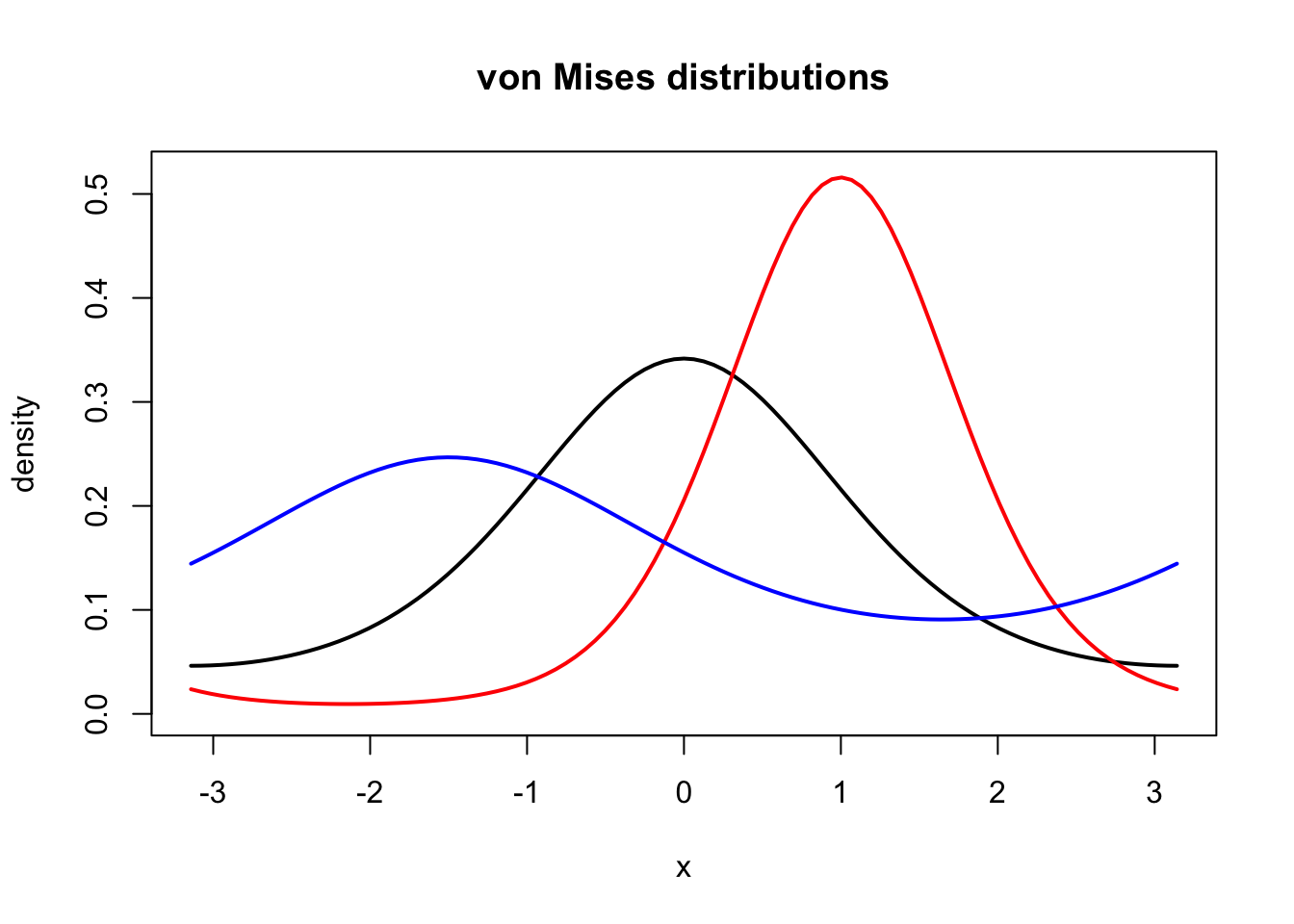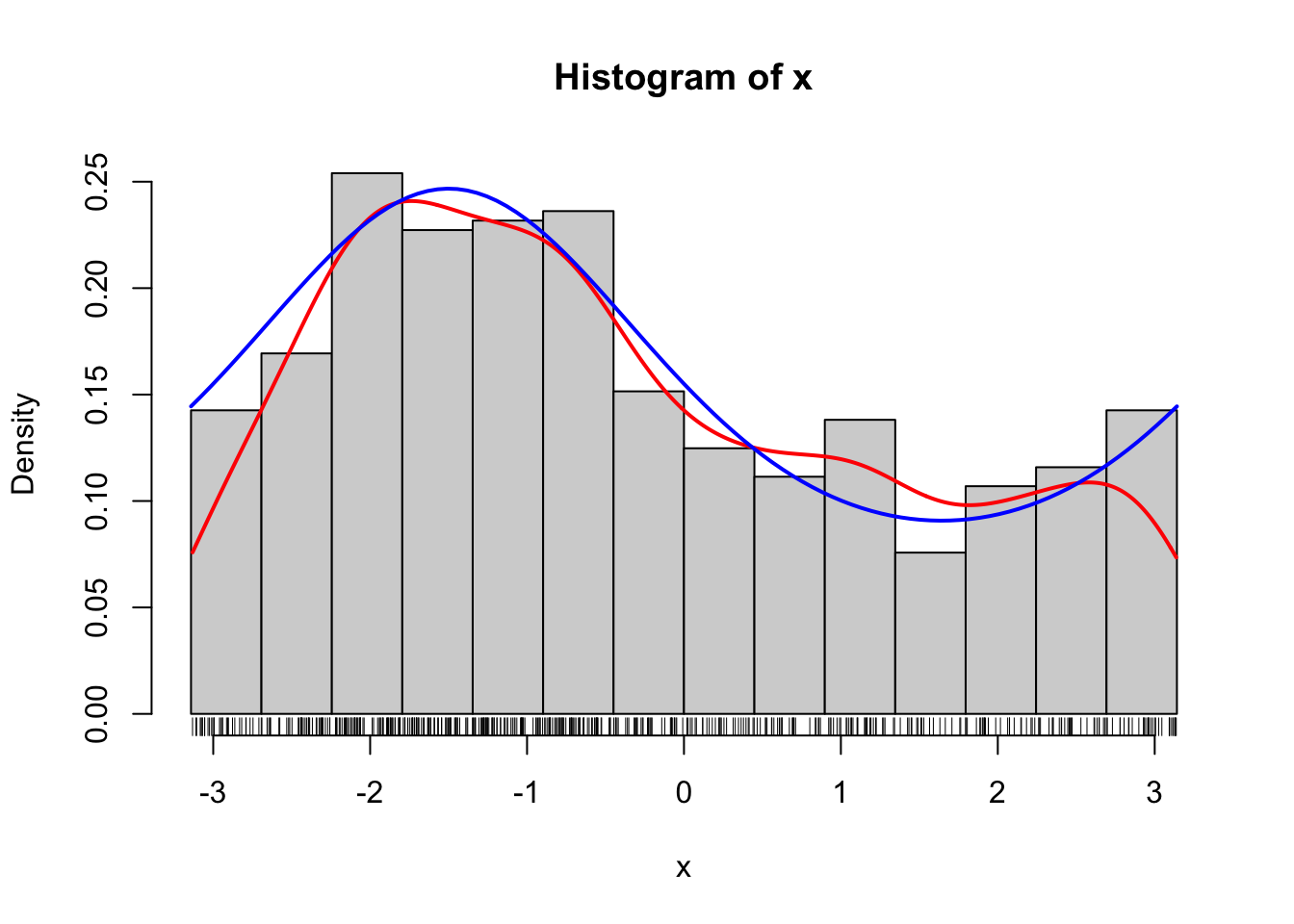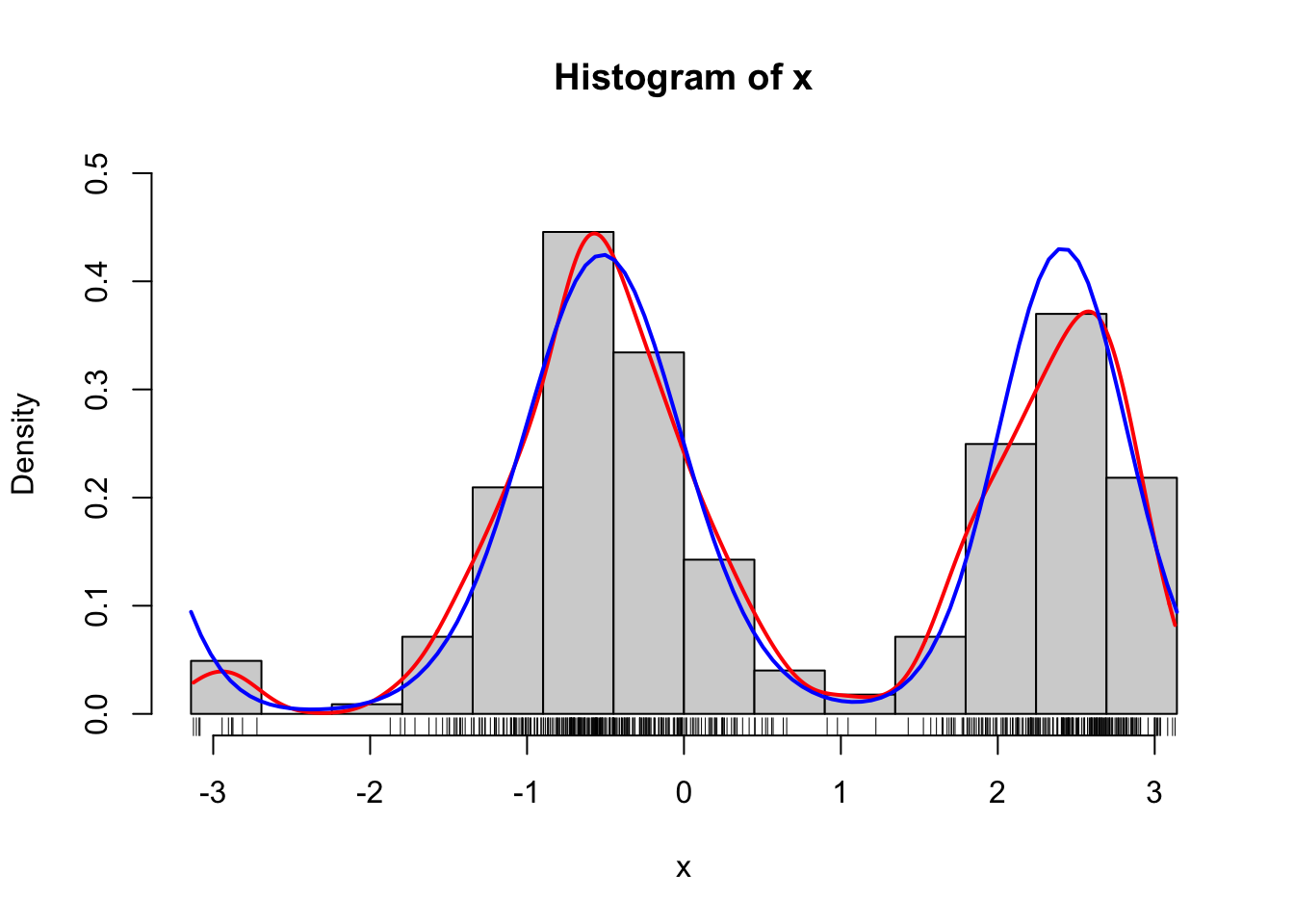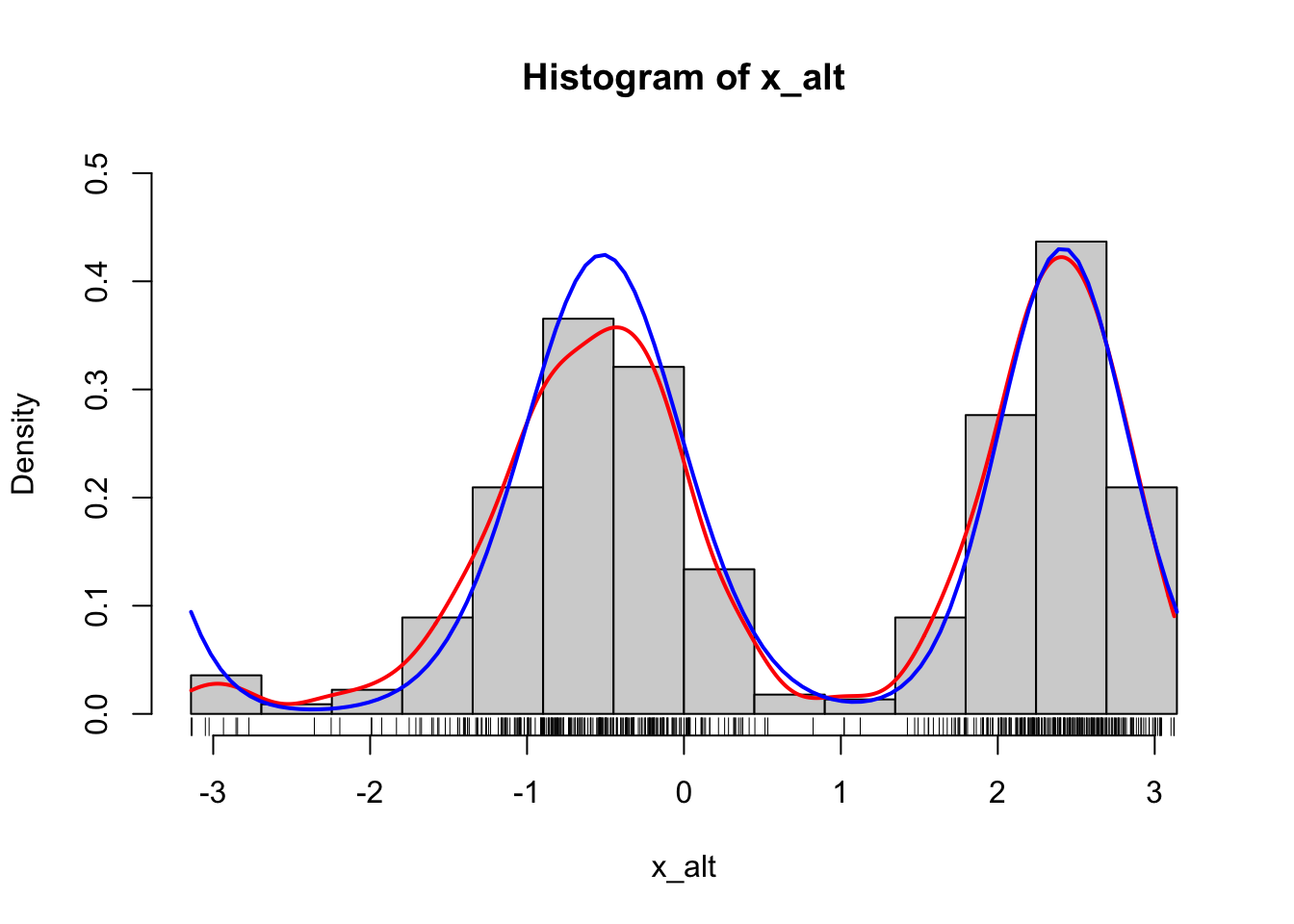## 1.2 Monte Carlo methods

Broadly speaking, Monte Carlo methods are computations that rely on some form of random input in order to carry out a computation. The actual random input will be generated by a (pseudo)random number generator according to some distributional specifications, and the precise value of the computation will depend on the precise value of the random input but in a way where we understand the magnitude of the dependence quite well. In most cases we can make the dependence diminish by increasing the amount of random input.

In statistics it is quite interesting in itself that we can make the computer simulate data so that we can draw an example data set from a statistical model. However, the real usage of such simulations is almost always as a part of a Monte Carlo computation, where we repeat the simulation of data sets a large number of times and compute distributional properties of various statistics. This is just one of the most obvious applications of Monte Carlo methods in statistics and there are many others. Disregarding the specific computation of interest in applications, a core problem of Monte Carlo methods is efficient simulation of random variables from a given target distribution.

### 1.2.1 Univariate von Mises distributions

We will exemplify Monte Carlo computations by considering angle distributions just as in Section 1.1. The angles take values in the interval $$(-\pi, \pi]$$, and we will consider models based on the von Mises distribution on this interval, which has density

$f(x) = \frac{1}{\varphi(\theta)} e^{\theta_1 \cos(x) + \theta_2 \sin(x)}$

for $$\theta = (\theta_1, \theta_2)^T \in \mathbb{R}^2$$. A common alternative parametrization is obtained by introducing $$\kappa = \|\theta\|_2 = \sqrt{\theta_1^2 + \theta_2^2}$$, and (whenever $$\kappa \neq 0$$) $$\nu = \theta / \kappa = (\cos(\mu), \sin(\mu))^T$$ for $$\mu \in (-\pi, \pi]$$. Using the $$(\kappa, \mu)$$-parametrization the density becomes
$f(x) = \frac{1}{\varphi(\kappa \nu)} e^{\kappa \cos(x - \mu)}.$ The former parametrization in terms of $$\theta$$ is, however, the canonical parametrization of the family of distributions as an exponential family, which is particularly useful for various likelihood estimation algorithms. The normalization constant

\begin{align*} \varphi(\kappa \nu) & = \int_{-\pi}^\pi e^{\kappa \cos(x - \mu)}\mathrm{d} x \\ & = 2 \pi \int_{0}^{1} e^{\kappa \cos(\pi x)}\mathrm{d} x = 2 \pi I_0(\kappa) \end{align*}

is given in terms of the modified Bessel function $$I_0$$. We can easily compute and plot the density using R’s besselI implementation of the modified Bessel function.

phi <- function(k) 2 * pi * besselI(k, 0)
curve(exp(cos(x)) / phi(1), -pi, pi, lwd = 2, ylab = "density", ylim = c(0, 0.52))
curve(exp(2 * cos(x - 1)) / phi(2), col = "red", lwd = 2, add = TRUE)
curve(exp(0.5 * cos(x + 1.5)) / phi(0.5), col = "blue", lwd = 2, add = TRUE)Figure 1.7: Density for the von Mises distribution with parameters $$\kappa = 1$$ and $$\nu = 0$$ (black), $$\kappa = 2$$ and $$\nu = 1$$ (red), and $$\kappa = 0.5$$ and $$\nu = - 1.5$$ (blue).

It is not entirely obvious how we should go about simulating data points from the von Mises distribution. It will be demonstrated in Section 4.3 how to implement a rejection sampler, which is one useful algorithm for simulating samples from a distribution with a density.

In this section we simply use the rmovMF function from the movMF package, which implements a few functions for working with (finite mixtures of) von Mises distributions, and even the general von Mises-Fisher distributions that are generalizations of the von Mises distribution to $$p$$-dimensional unit spheres.

library("movMF")
xy <- rmovMF(500, 0.5 * c(cos(-1.5), sin(-1.5)))
# rmovMF represents samples as elements on the unit circle
x <- acos(xy[, 1]) * sign(xy[, 2])
hist(x, breaks = seq(-pi, pi, length.out = 15), prob = TRUE)
rug(x)
density(x, bw = "SJ", cut = 0) %>% lines(col = "red", lwd = 2)
curve(exp(0.5 * cos(x + 1.5)) / phi(0.5), col = "blue", lwd = 2, add = TRUE)Figure 1.8: Histogram of 500 simulated data points from a von Mises distribution with parameters $$\kappa = 0.5$$ and $$\nu = - 1.5$$. A smoothed density estimate (red) and the true density (blue) are added to the plot.

### 1.2.2 Mixtures of von Mises distributions

The von Mises distributions are unimodal distributions on $$(-\pi, \pi]$$. Thus to find a good model of the bimodal angle data, say, we have do move beyond these distributions. A standard approach for constructing multimodal distributions is as mixtures of unimodal distributions. A mixture of two von Mises distributions can be constructed by flipping a (biased) coin to decide which of the two distributions to sample from. We will use the exponential family parametrization in the following.

thetaA <- c(3.5, -2)
thetaB <- c(-4.5, 4)
alpha <- 0.55 # Probability of von Mises distribution A
# The sample function implements the "coin flips"
u <- sample(c(1, 0), 500, replace = TRUE, prob = c(alpha, 1 - alpha))
xy <- rmovMF(500, thetaA) * u + rmovMF(500, thetaB) * (1 - u)
x <- acos(xy[, 1]) * sign(xy[, 2])

The rmovMF actually implements simulation from a mixture distribution directly, thus there is no need to construct the “coin flips” explicitly.

theta <- rbind(thetaA, thetaB)
xy <- rmovMF(length(x), theta, c(alpha, 1 - alpha))
x_alt <- acos(xy[, 1]) * sign(xy[, 2])

To compare the simulated data with two mixture components to the model and a smoothed density, we implement an R function that computes the density for an angle argument using the function dmovMF that takes a unit circle argument.

dvM <- function(x, theta, alpha) {
xx <- cbind(cos(x), sin(x))
dmovMF(xx, theta, c(alpha, 1 - alpha)) / (2 * pi)
}

Note that dmovMF uses normalized spherical measure on the unit circle as reference measure, thus the need for the $$2\pi$$ division if we want the result to be comparable to histograms and density estimates that use Lebesgue measure on $$(-\pi, \pi]$$ as the reference measure.

hist(x, breaks = seq(-pi, pi, length.out = 15), prob = TRUE, ylim = c(0, 0.5))
rug(x)
density(x, bw = "SJ", cut = 0) %>% lines(col = "red", lwd = 2)
curve(dvM(x, theta, alpha), col = "blue", lwd = 2, add = TRUE)

hist(x_alt, breaks = seq(-pi, pi, length.out = 15), prob = TRUE, ylim = c(0, 0.5))
rug(x_alt)
density(x_alt, bw = "SJ", cut = 0) %>% lines(col = "red", lwd = 2)
curve(dvM(x, theta, alpha), col = "blue", lwd = 2, add = TRUE)Figure 1.9: Histograms of 500 simulated data points from a mixture of two von Mises distributions using either the explicit construction of the mixture (left) or the functionality in rmovMF to simulate mixtures directly (right). A smoothed density estimate (red) and the true density (blue) are added to the plot.

Simulation of data from a distribution finds many applications. The technique is widely used whenever we want to investigate a statistical methodology in terms of its frequentistic performance under various data sampling models, and simulation is a tool of fundamental importance for the practical application of Bayesian statistical methods. Another important application is as a tool for computing approximations of integrals. This is usually called Monte Carlo integration and is a form of numerical integration. Computing probabilities or distribution functions, say, are notable examples of integrals, and we consider here the computation of the probability of the interval $$(0, 1)$$ for the above mixture of two von Mises distributions.

It is straightforward to compute this probability via Monte Carlo integration as a simple average. Note that we will use a large number of samples, 50,000 in this case, of simulated angles for this computation. Increasing the number even further will make the result more accurate. Chapter 5 deals with the assessment of the accuracy of Monte Carlo integrals, and how this random error can be estimated, bounded and minimized.

xy <- rmovMF(50000, theta, c(alpha, 1 - alpha))
x <- acos(xy[, 1]) * sign(xy[, 2])
mean(x > 0 & x < 1)  # Estimate of the probability of the interval (0, 1)
##  0.08662

The probability above could, of course, be expressed using the distribution function of the mixture of von Mises distributions, which in turn can be computed in terms of integrals of von Mises densities. Specifically, the probability is $p = \frac{\alpha}{\varphi(\theta_A)} \int_0^1 e^{\theta_{A, 1} \cos(x) + \theta_{A, 2} \sin(x)} \mathrm{d} x + \frac{1 - \alpha}{\varphi(\theta_B)} \int_0^1 e^{\theta_{B, 1} \cos(x) + \theta_{B, 2} \sin(x)} \mathrm{d} x,$ but these integrals do not have a simple analytic representation – just as the distribution function of the von Mises distribution doesn’t have a simple analytic expression. Thus the computation of the probability requires numerical computation of the integrals.

The R function integrate can be used for numerical integration of univariate functions using standard numerical integration techniques. We can thus compute the probability by integrating the density of the mixture, as implemented above as the R function dvM. Note the arguments passed to integrate below. The first argument is the density function, then follows the lower and the upper limits of the integration, and then follows additional arguments to the density – in this case parameter values.

integrate(dvM, 0, 1, theta = theta, alpha = alpha)
## 0.08635171 with absolute error < 9.6e-16

The integrate function in R is an interface to a couple of classical QUADPACK Fortran routines for numerical integration via adaptive quadrature. Specifically, the computations rely on approximations of the form $\int_a^b f(x) \mathrm{d} x \simeq \sum_i w_i f(x_i)$ for certain grid points $$x_i$$ and weights $$w_i$$, which are computed using Gauss-Kronrod quadrature. This method provides an estimate of the approximation error in addition to the numerical approximation of the integral itself.

It is noteworthy that integrate as a function implemented in R takes another function, in this case the density dvM, as an argument. R is a functional programming language and functions are first-class citizens. This implies, for instance, that functions can be passed as arguments to other functions using a variable name – just like any other variable can be passed as an argument to a function. In the parlance of functional programming, integrate is a functional: a higher-order function that takes a function as argument and returns a numerical value. One of the themes of this book is how to make good use of functional (and object oriented) programming features in R to write clear, expressive and modular code without sacrificing computational efficiency.

Returning to the specific problem of the computation of an integral, we may ask what the purpose of Monte Carlo integration is? Apparently we can just do numerical integration using e.g. integrate. There are at least two reasons why Monte Carlo integration is sometimes preferable. First, it is straightforward to implement and often works quite well for multivariate and even high-dimensional integrals, whereas grid-based numerical integration schemes scale poorly with the dimension. Second, it does not require that we have an analytic representation of the density. It is common in statistical applications that we are interested in the distribution of a statistic, which is a complicated transformation of data, and whose density is difficult or impossible to find analytically. Yet if we can just simulate data, we can simulate from the distribution of the statistic, and we can then use Monte Carlo integration to compute whatever probability or integral w.r.t. the distribution of the statistic that we are interested in.

### 1.2.3 Large scale Monte Carlo methods

Monte Carlo methods are used pervasively in statistics and in many other sciences today. It is nowadays trivial to simulate millions of data points from a simple univariate distribution like the von Mises distribution, and Monte Carlo methods are generally attractive because they allow us to solve computational problems approximately in many cases where exact or analytic computations are impossible and alternative deterministic numerical computations require more adaptation to the specific problem.

Monte Carlo methods are thus really good off-the-shelf methods, but scaling the methods up can be a challenge and is a contemporary research topic. For the simulation of multivariate $$p$$-dimensional data it can, for instance, make a big difference whether the algorithms scale linearly in $$p$$ or like $$O(p^a)$$ for some $$a > 1$$. Likewise, some Monte Carlo methods (e.g.  Bayesian computations) depend on a data set of size $$n$$, and for large scale data sets, algorithms that scale like $$O(n)$$ are really the only algorithms that can be used in practice.